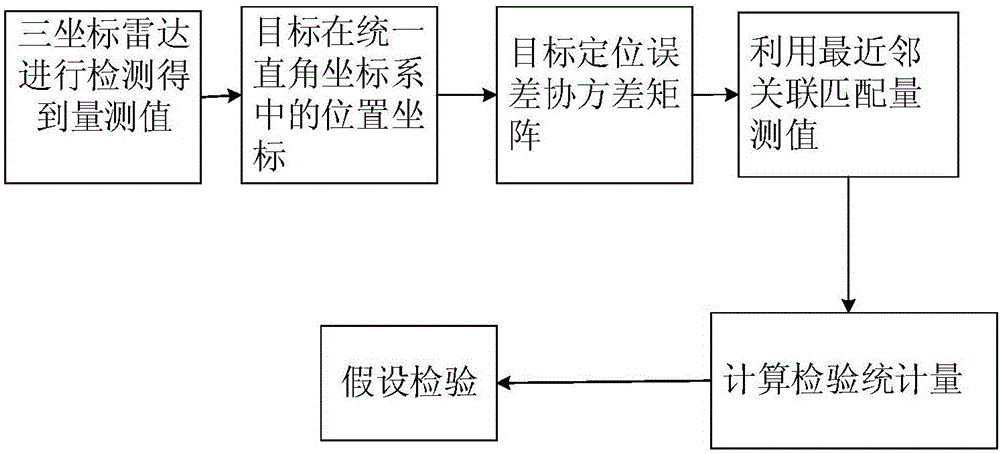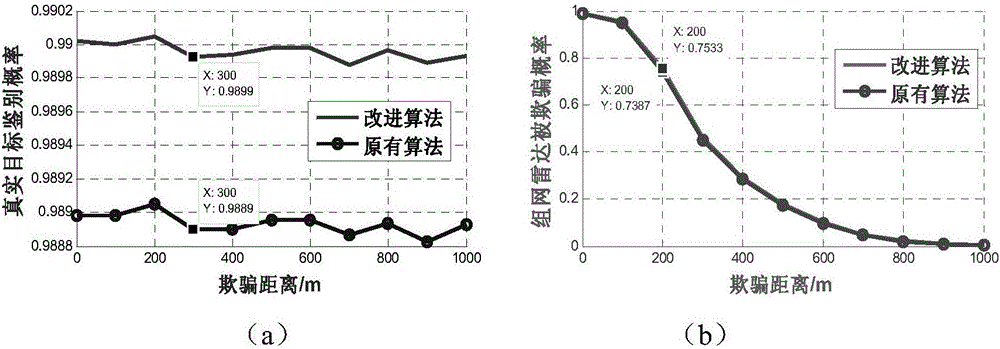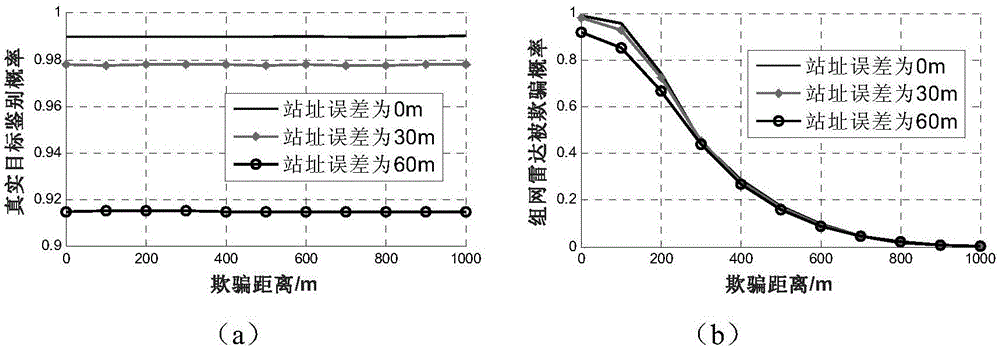# Method for withstanding false targets on basis of station's position error fusion algorithm

## A fusion algorithm and false target technology, applied in radio wave measurement systems, instruments, etc., can solve the problems of radar station accuracy, affecting the identification of true and false targets, and large positioning errors, so as to reduce resource consumption, reduce the probability of being deceived, The effect of increasing the reference value

Active Publication Date: 2017-05-17
XIDIAN UNIV
4 Cites 7 Cited by

## AI-Extracted Technical Summary

### Problems solved by technology

However, the original algorithm ignored the actual radar site error in the modeling process, that is, the estimation error of the radar station’s own position: the radar station’s own positioning cannot be extremely accurat...
View more

## Abstract

The invention discloses a method for withstanding false targets on the basis of a station's position error fusion algorithm, and mainly solves the problems that station's position error factors are not considered when false targets are withstand in the prior art, so that detection results are inaccurate. According to the scheme, the method comprises the following steps: 1, measuring a target by a node radar; 2, carrying out coordinates transformation on measured values to obtain the position information of the target in a uniform rectangular coordinate system; 3, calculating to obtain a positioning error covariance matrix of the target through the position information of the target in the uniform rectangular coordinate system; 4, matching the measured values by a nearest neighbor association method to obtain an associated measurement sequences; and 5, combining the measured values in all the associated measurement sequences in pairs, calculating the mahalanobis distance between each two measured values through the positioning error covariance matrix of the target, carrying out hypothesis testing on the mahalanobis distances, and getting rid of false targets to finish identification of real targets and false targets. By the method, the correct identification probability of the rear targets is improved, and therefore, the method can be used for detecting or tracking targets.

Application Domain

Wave based measurement systems

Technology Topic

Position errorNear neighbor +7

## Image

•••## Examples

• Experimental program(1)

### Example Embodiment

 refer to figure 1 , the concrete realization steps of the present invention are as follows:
 Step 1, the networked radar measures the target.
 Each node radar in the networked radar system measures the target separately, and the measurement value of the i-th node radar for the k-th target is in, is the radial distance of the k-th target relative to the i-th node radar, is the azimuth of the k-th target relative to the i-th node radar, is the pitch angle of the k-th target relative to the i-th node radar;
 Step 2, coordinate transformation
 Perform coordinate transformation on the measured value of the target obtained in (1) to obtain the coordinates of the target in the unified rectangular coordinate system in:

 In the formula, are the radial distance, azimuth and pitch information of the target relative to the node radar, respectively, are the x-axis, y-axis, and z-axis coordinates of the i-th node radar in the unified coordinate system, respectively.
 Step 3: Derive the positioning error covariance matrix.
 3a) For the position coordinate M of the target in the unified rectangular coordinate system i (k) Take the derivation to obtain the relationship expression between the target positioning position error, the radar measurement error, and the site error:

 in, are the radial distance, azimuth angle and pitch angle information of the target relative to the node radar, respectively;
 3b) The target positioning error Denoted as dX, the radar measurement error Denote it as dV, and denote the radar site error as dX s , the relationship between the target positioning position error, radar measurement error, and site error in 3a) is simplified as:
 dX=CdV+dX s
 in,

 From the above relationship, when the target position information is transformed into a unified rectangular coordinate system, the target positioning error dX, the radar measurement error dV and the radar site error dX s In a linear relationship, the Gaussian distribution is still valid, so the target positioning error dX is still a Gaussian distribution random variable with zero mean;
 3c) Calculate the target positioning error covariance matrix P:
 P=E[dXdX T ]
 =CE[dVdV T ]C T +E[dX s dX s T ]
 in,


 σ r , σ θ , are the ranging error, azimuth angle error and pitch angle error of the radar, respectively; σ xs , σ ys , σ zs are the site errors of the radar on the x-axis, y-axis and z-axis, respectively.
 Step 4: Obtain an association measurement sequence through nearest neighbor association.
 The data in the measurement set of each node radar is matched by the method of nearest neighbor correlation, and the measurement value with the smallest Euclidean distance is matched to the same correlation measurement sequence. One correlation measurement sequence corresponds to the amount of a target in different node radars. Measured value, its realization steps are as follows:
 4a) Find the node radar s with the least measured value as the reference radar;
 4b) Select a measurement value s of the reference radar s i , calculate its Euclidean distance from all measurements of the l-th radar:
 in, are the x-axis, y-axis, and z-axis coordinates of the i-th measurement value of the reference radar s, is the x-axis, y-axis, and z-axis coordinates of the jth measurement value of node radar 1;
 4c) Select the measurement value of the lth radar corresponding to the minimum value of the Euclidean distance obtained in 4b) to match the measurement value of the reference radar s to the same associated measurement sequence.
 Step 5, perform hypothesis testing.
 5a) Propose two mutually opposing first hypotheses H 0 and the second hypothesis H 1 :
 The first hypothesis H 0 for the two measurements Z i and Z j Generated in different node radars for real targets;
 Second Hypothesis H 1 for the two measurements Z i and Zj At least one of them is generated by deceptive false targets;
 5b) Calculate the difference between any two radar measurement errors ΔZ=dZ i -dZ j ~N(0,∑ ij ),in,
 ∑ ij =E[(dZ i- dZ j )(dZ i- dZ j ) T ]=P i +P j
 dZ i is the measurement error of the i-th measurement value, dZ j is the measurement error of the jth measurement value, then in H 0 Under the condition that is established, ΔZ also approximately obeys the zero-mean Gaussian distribution;
 5c) Calculate the Mahalanobis distance between the radar measurements of two nodes in an association sequence:

 Among them, Z i is the i-th measurement value, Z j For the jth measurement value, the Mahalanobis distance d ij As a statistical test quantity, in H 0 Under the condition that is established, the Mahalanobis distance d ij Obey the distribution of the other party, so according to the δ of the decision threshold given by the radar user, the Mahalanobis distance d ij Do the following hypothesis tests:
 if d ij ≤δ is established, then accept the first hypothesis H 0 , that is, to determine the two measurement values ​​Z i and Z j is the measurement value of the real target in different node radars;
 if d ijδ is established, then accept the second hypothesis H 1 , that is, to determine the two measurement values ​​Z i and Z j For the measurement values ​​of false targets in different node radars, the identification of true and false targets is completed.
 The effectiveness of the present invention against deceptive false targets can be verified by the following simulation experiments.
 1. Experimental scenario: three radars are used as the networking model of the node radar, and a real target is set, whose position is 50km in the x-dimension direction, 50km in the y-dimension direction, and 50km in the z-dimension direction. The coordinates are 1km, and a deceptive false target is set for each radar station. The radar information of each node is shown in Table 1. Among them, the positioning error of the node radar to itself is the same in three dimensions, namely In the hypothesis testing model, the significance level α=0.01, and the detection threshold δ=9.21.
 Table 1 Radar parameter information table of each node

 2. Experiment content and result analysis
 Experiment 1: Change the deception distance of the false target, and the variation range is 0m to 1000m. Use the present invention and the existing networked radar anti-deceptive false target jamming algorithm to distinguish the true and false targets respectively, and obtain the correct identification probability and networking of the true target. The probability of radar being deceived, the results are as follows figure 2 shown. in figure 2 (a) is the variation curve of the discrimination probability of the true target, figure 2 (b) is the change curve of the probability of being deceived by the networked radar.
 Depend on figure 2 (a) it can be seen: the correct identification probability of the present invention to the true target is higher than the correct identification probability of the existing algorithm to the true target, and along with the increase of the false target deception distance, the correct identification probability of the two algorithms to the true target is basically unchanged; by figure 2 (b) It can be seen that with the increase of the false target deception distance, the deception probability of the networked radar obtained by the two algorithms gradually decreases, and the deception probability of the networked radar obtained by the original algorithm is slightly lower than that in the present invention. The probability of being deceived by the network radar obtained by the algorithm.
 Experiment 2: Change the deception distance of false targets, ranging from 0m to 1000m, use the invention to identify true and false targets, set three groups of different radar site errors, 0m, 30m, 60m, respectively, and count the real target identification Changes in the probability and the probability of being deceived by the networked radar, the results are as follows image 3 shown, where image 3 (a) is the variation curve of the discrimination probability of the true target, image 3 (b) is the change curve of the probability of being deceived by the networked radar.
 from image 3 (a) It can be seen that when using the present invention to identify true and false targets, the size of the radar site error affects the real target identification probability and the probability of being deceived by the networked radar. With the increase of the site error, the real target identification probability gradually decreases. The probability of being deceived by the networked radar is gradually reduced. Therefore, after considering the site error factor, the detection probability and the deception probability obtained by the original algorithm can be corrected, bringing more accurate data to real applications and avoiding unnecessary resource consumption.

## PUM## Description & Claims & Application Information

We can also present the details of the Description, Claims and Application information to help users get a comprehensive understanding of the technical details of the patent, such as background art, summary of invention, brief description of drawings, description of embodiments, and other original content. On the other hand, users can also determine the specific scope of protection of the technology through the list of claims; as well as understand the changes in the life cycle of the technology with the presentation of the patent timeline. Login to view more.

## Prediction method for electromagnetic radiation of CDMA base station

ActiveCN108229757AEfficient and Precise PredictionHigh reference value
Owner:XIANGTAN UNIV

## Classification method and device

InactiveCN109165677AHigh reference value
Owner:BEIJING FRIENDSHIP HOSPITAL CAPITAL MEDICAL UNIV

## Metal material heat shrinkage parameter measuring device used for 3D printing and analogue simulation

InactiveCN109521045Aflexible operationHigh reference value
Owner:广西慧思通科技有限公司

## Representative verbal skill fragment extraction device and method based on seat voice segmentation

PendingCN111475634AHigh reference valueSave time on self-improvement and learning
Owner:FUDAN UNIV

## A long-term water stability evaluation test device and method of asphalt mixture

ActiveCN110873669BImprove applicabilityHigh reference value
Owner:JIANGSU ZHONGLU ENG DETECTION CO LTD

## Classification and recommendation of technical efficacy words

• Reduce the chance of being cheated
• High reference value

## Anti-counterfeiting ticket

InactiveCN104517523Areduce the chance of being cheated
Owner:BEIJING HONGMA MEDIA CULTURE DEV
Who we serve
• R&D Engineer
• R&D Manager
• IP Professional
Why Eureka